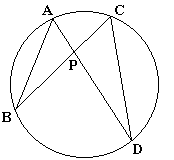The Intersecting Chords Theorem asserts the following:Given a point P in the interior of a circle, pass two lines through P that intersect the circle in points A and D and, respectively, B and C. Then AP·DP = BP·CP.

The proof follows easily from the similarity of triangles ABP and CDP that is a consequence of the equality of their angles:BAD =BCD, as inscribed angles subtended by the same chord BD,ABC =ADC, as inscribed angles subtended by the same chord AC,APB =CPD, as a pair of vertical angles.

(Vertical angles are formed by the same intersecting lines, but the opposite rays - the halves of the lines - cut off by their point of intersection.)

From the similarity of triangles ABP and CDP, we obtain the proportion:

 (*) AP/CP = BP/DP = AB/CD.

The first identity (AP/CP = BP/DP) leads directly to the Intersecting Chords Theorem: AP·DP = BP·CP.

Q.E.D.